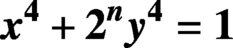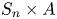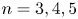Home

### Diophantine equations (11Dxx)

• Article
• ##### THE DIOPHANTINE EQUATION$\boldsymbol{x}^{\boldsymbol{4}} \boldsymbol{+} \boldsymbol{2}^{\boldsymbol{n}}\boldsymbol{y}^{\boldsymbol{4}} \boldsymbol{=} \boldsymbol{1}$ IN QUADRATIC NUMBER FIELDS
• Bulletin of the Australian Mathematical Society, Volume 104, Issue 1

• Article
• ##### THE EXPONENTIAL DIOPHANTINE PROBLEM FOR${\mathbb {Q}}$
• The Journal of Symbolic Logic, Volume 85, Issue 2

### Forms and linear algebraic groups (11Exx)

• Article
• ##### A proof of the linear Arithmetic Fundamental Lemma for$\operatorname {{\mathrm {GL}}}_4$
• Canadian Journal of Mathematics, First View

### Probabilistic theory: distribution modulo $1$; metric theory of algorithms (11Kxx)

• Article
• ##### On the triple correlations of fractional parts of$n^2\alpha$
• Canadian Journal of Mathematics, First View

### Exponential sums and character sums (11Lxx)

• Article
• ##### On the triple correlations of fractional parts of$n^2\alpha$
• Canadian Journal of Mathematics, First View

### Zeta and $L$-functions: analytic theory (11Mxx)

• Article
• ##### ANALYTIC PROPERTIES OF EISENSTEIN SERIES AND STANDARD$L$ -FUNCTIONS
• Nagoya Mathematical Journal, First View

• Article
• ##### Vanishing of multizeta values over$\mathbb {F}_q[t]$ at negative integers
• Canadian Mathematical Bulletin, First View

### Additive number theory; partitions (11Pxx)

• Article
• ##### DIVISIBILITY OF CERTAIN SINGULAR OVERPARTITIONS BY POWERS OF$\textbf{2}$ AND$\textbf{3}$
• Bulletin of the Australian Mathematical Society, First View

### Algebraic number theory: global fields (11Rxx)

• Article
• ##### Vanishing of multizeta values over$\mathbb {F}_q[t]$ at negative integers
• Canadian Mathematical Bulletin, First View

• Article
• ##### Malle's conjecture for$S_n\times A$ for$n = 3,4,5$
• Compositio Mathematica, Volume 157, Issue 1

### Algebraic number theory: local and $p$-adic fields (11Sxx)

• Article
• ##### The epsilon constant conjecture for higher dimensional unramified twists of${\mathbb Z}_p^r$ (1)
• Canadian Journal of Mathematics, First View

• Article
• ##### CHARACTERIZING THE MOD-$\ell$ LOCAL LANGLANDS CORRESPONDENCE BY NILPOTENT GAMMA FACTORS
• Nagoya Mathematical Journal, First View

### Connections with logic (11Uxx)

• Article
• ##### WAVE FRONT HOLONOMICITY OF$\text{C}^{\text{exp}}$ -CLASS DISTRIBUTIONS ON NON-ARCHIMEDEAN LOCAL FIELDS
• Forum of Mathematics, Sigma, Volume 8